# Resources tagged with: Addition & subtraction

Filter by: Content type:
Age range:
Challenge level:

There are 227 NRICH Mathematical resources connected to Addition & subtraction, you may find related items under Calculations and Numerical Methods.##### Age 5 to 11Challenge Level

Try out this number trick. What happens with different starting numbers? What do you notice?### Can You Make 100?

##### Age 11 to 14Challenge Level

How many ways can you find to put in operation signs (+ - x Ã·) to make 100?### Subtraction Surprise

##### Age 7 to 14Challenge Level

Try out some calculations. Are you surprised by the results?### Making Sense of Positives and Negatives

##### Age 11 to 14

This article suggests some ways of making sense of calculations involving positive and negative numbers.### Take Three Numbers

##### Age 7 to 11Challenge Level

What happens when you add three numbers together? Will your answer be odd or even? How do you know?### Dotty Six

##### Age 5 to 11Challenge Level

Dotty Six is a simple dice game that you can adapt in many ways.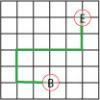### Journeys in Numberland

##### Age 7 to 11Challenge Level

Tom and Ben visited Numberland. Use the maps to work out the number of points each of their routes scores.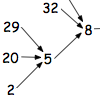##### Age 7 to 11Challenge Level

What happens when you add the digits of a number then multiply the result by 2 and you keep doing this? You could try for different numbers and different rules.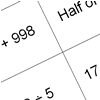### How Do You Do It?

##### Age 7 to 11Challenge Level

This group activity will encourage you to share calculation strategies and to think about which strategy might be the most efficient.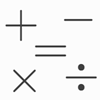### Which Symbol?

##### Age 7 to 11Challenge Level

Choose a symbol to put into the number sentence.### Nice or Nasty

##### Age 7 to 14Challenge Level

There are nasty versions of this dice game but we'll start with the nice ones...### Countdown Fractions

##### Age 11 to 16Challenge Level

Here is a chance to play a fractions version of the classic Countdown Game.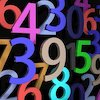### Countdown

##### Age 7 to 14Challenge Level

Here is a chance to play a version of the classic Countdown Game.### Magic Vs

##### Age 7 to 11Challenge Level

Can you put the numbers 1-5 in the V shape so that both 'arms' have the same total?### Crossed Ends

##### Age 11 to 14Challenge Level

Crosses can be drawn on number grids of various sizes. What do you notice when you add opposite ends?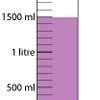### Oh! Harry!

##### Age 7 to 11Challenge Level

A group of children are using measuring cylinders but they lose the labels. Can you help relabel them?### Weights

##### Age 11 to 14Challenge Level

Different combinations of the weights available allow you to make different totals. Which totals can you make?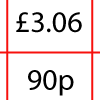### How Much Did it Cost?

##### Age 7 to 11Challenge Level

Use your logical-thinking skills to deduce how much Dan's crisps and ice-cream cost altogether.### Adding and Subtracting Positive and Negative Numbers

##### Age 11 to 14

How can we help students make sense of addition and subtraction of negative numbers?### Sea Level

##### Age 7 to 11Challenge Level

The picture shows a lighthouse and many underwater creatures. If you know the markings on the lighthouse are 1m apart, can you work out the distances between some of the different creatures?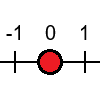### Tug Harder!

##### Age 7 to 11Challenge Level

In this game, you can add, subtract, multiply or divide the numbers on the dice. Which will you do so that you get to the end of the number line first?### Consecutive Negative Numbers

##### Age 11 to 14Challenge Level

Do you notice anything about the solutions when you add and/or subtract consecutive negative numbers?### First Connect Three

##### Age 7 to 11Challenge Level

Add or subtract the two numbers on the spinners and try to complete a row of three. Are there some numbers that are good to aim for?### Connect Three

##### Age 11 to 16Challenge Level

In this game the winner is the first to complete a row of three. Are some squares easier to land on than others?### Cunning Card Trick

##### Age 11 to 14Challenge Level

Delight your friends with this cunning trick! Can you explain how it works?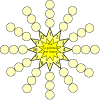### The Twelve Pointed Star Game

##### Age 7 to 11Challenge Level

Have a go at this game which involves throwing two dice and adding their totals. Where should you place your counters to be more likely to win?### Diagonal Sums

##### Age 7 to 14Challenge Level

In this 100 square, look at the green square which contains the numbers 2, 3, 12 and 13. What is the sum of the numbers that are diagonally opposite each other? What do you notice?### Number Differences

##### Age 7 to 11Challenge Level

Place the numbers from 1 to 9 in the squares below so that the difference between joined squares is odd. How many different ways can you do this?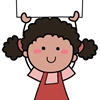### Carrying Cards

##### Age 7 to 11Challenge Level

These sixteen children are standing in four lines of four, one behind the other. They are each holding a card with a number on it. Can you work out the missing numbers?### Consecutive Seven

##### Age 11 to 14Challenge Level

Can you arrange these numbers into 7 subsets, each of three numbers, so that when the numbers in each are added together, they make seven consecutive numbers?### Number Pyramids

##### Age 11 to 14Challenge Level

Try entering different sets of numbers in the number pyramids. How does the total at the top change?### A Mixed-up Clock

##### Age 7 to 11Challenge Level

There is a clock-face where the numbers have become all mixed up. Can you find out where all the numbers have got to from these ten statements?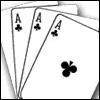### Amazing Card Trick

##### Age 11 to 14Challenge Level

How is it possible to predict the card?### The Deca Tree

##### Age 7 to 11Challenge Level

Find out what a Deca Tree is and then work out how many leaves there will be after the woodcutter has cut off a trunk, a branch, a twig and a leaf.### A Square of Numbers

##### Age 7 to 11Challenge Level

Can you put the numbers 1 to 8 into the circles so that the four calculations are correct?### Cinema Problem

##### Age 11 to 14Challenge Level

A cinema has 100 seats. Show how it is possible to sell exactly 100 tickets and take exactly Â£100 if the prices are Â£10 for adults, 50p for pensioners and 10p for children.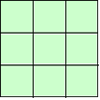### 1, 2, 3 Magic Square

##### Age 7 to 11Challenge Level

Arrange three 1s, three 2s and three 3s in this square so that every row, column and diagonal adds to the same total.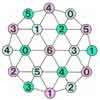### Totality

##### Age 5 to 11Challenge Level

This is an adding game for two players.### Cubes Within Cubes

##### Age 7 to 14Challenge Level

We start with one yellow cube and build around it to make a 3x3x3 cube with red cubes. Then we build around that red cube with blue cubes and so on. How many cubes of each colour have we used?### Two Primes Make One Square

##### Age 7 to 11Challenge Level

Can you make square numbers by adding two prime numbers together?### One Wasn't Square

##### Age 7 to 11Challenge Level

Mrs Morgan, the class's teacher, pinned numbers onto the backs of three children. Use the information to find out what the three numbers were.### Abundant Numbers

##### Age 7 to 11Challenge Level

48 is called an abundant number because it is less than the sum of its factors (without itself). Can you find some more abundant numbers?### Zios and Zepts

##### Age 7 to 11Challenge Level

On the planet Vuv there are two sorts of creatures. The Zios have 3 legs and the Zepts have 7 legs. The great planetary explorer Nico counted 52 legs. How many Zios and how many Zepts were there?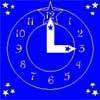### Watch the Clock

##### Age 7 to 11Challenge Level

During the third hour after midnight the hands on a clock point in the same direction (so one hand is over the top of the other). At what time, to the nearest second, does this happen?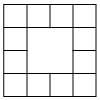### Prison Cells

##### Age 7 to 11Challenge Level

There are 78 prisoners in a square cell block of twelve cells. The clever prison warder arranged them so there were 25 along each wall of the prison block. How did he do it?### Number Daisy

##### Age 11 to 14Challenge Level

Can you find six numbers to go in the Daisy from which you can make all the numbers from 1 to a number bigger than 25?### Two and Two

##### Age 11 to 16Challenge Level

How many solutions can you find to this sum? Each of the different letters stands for a different number.### Neighbours

##### Age 7 to 11Challenge Level

In a square in which the houses are evenly spaced, numbers 3 and 10 are opposite each other. What is the smallest and what is the largest possible number of houses in the square?### Summing Consecutive Numbers

##### Age 11 to 14Challenge Level

15 = 7 + 8 and 10 = 1 + 2 + 3 + 4. Can you say which numbers can be expressed as the sum of two or more consecutive integers?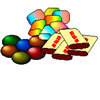### The Puzzling Sweet Shop

##### Age 5 to 11Challenge Level

There were chews for 2p, mini eggs for 3p, Chocko bars for 5p and lollypops for 7p in the sweet shop. What could each of the children buy with their money?